Next: CLEAR Up: Commands Previous: WRITEPRINT

## PLOT

Plot using TopDrawer.

PLOT HIST, [NONEWPAGE,] [RIGHTLEFT,] [KIND=k(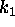,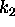),] [GENERATION rel n,] [INCP,] H=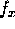, [HSCALE=(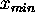,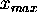,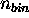),] [HLOG,] [VLOG,] [TITLE='head_title',] [HTITLE='bottom_title',] [VTITLE='left_title',] [FILE=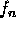,] ;

NONEWPAGE
Do not insert `NEWFRAME' of TopDrawer so that the figure is written on the previous plot on the same file. This makes sense when the new plot has the same scale as the previous plot.
RIGHTLEFT
Select right(left)-going particles only.
k,,Select photons (k=1), electrons (k=2), positrons (k=3) only.
rel
Relational operator.   One of =, &#;'74, &#;'76, &#;'74=, &#;'76=, =&#;'74, =&#;'76, &#;'74, &#;'76.
n
Generation.  Select particles whose generation satisfies the relation ``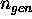rel n''.
INCP
Include particles created by incoherent incoherent processes only. Otherwise normal particles only.An expression defining the horizontal variable. Following running variables  can be used. (See Sec.2.4)
T, X, Y, S, En, Px, Py, Ps, Sx, Sy, Ss, Xi1, Xi2, Xi3, Kind, Gen
For example,
H =Sqrt[(Px2+Py2)/Ps2]*1E6
defines the orbit angle in micro-radians.,,Minimum and maximum of the horizontal scale and the number of bins. If omitted, the minimum and maximum in the particle data are used for,and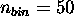.
HLOG,VLOG
Log scale of horizontal and vertical axes. When HLOG is specified,andmust be specified explicitly. The binning interval will be equal in log-scale.
top_title, etc
Title string. Must be enclosed by a pair of apostrophes. Topdrawer case string can be specified by using ``;'' as the delimitor like
TITLE='E0G1; XGX;', for writing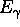. It is recommended to put ``;'' also at the end, as in this example, to avoid writing unnecessary blanck characters.Output file reference number. Default=TDFile.

PLOT SCATTER, [NONEWPAGE,] [RIGHTLEFT,] [KIND=k(,),] [GENERATION rel n,] [INCP,] H=, V=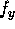, [HSCALE=(,),] [VSCALE=(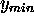,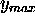),] [HLOG,] [VLOG,] [MAXNP=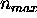,] [TITLE='top_title',] [HTITLE='bottom_title',] [VTITLE='left_title',] [FILE=,] ;An expression defining the vertical variable.,Minimum and maximum of the vertical scale.Maximum number of points to be plotted (in order to save the plotting time). Randomly selected. Default: plot all points.

Other operands are the same as for the histogram.

PLOT TESTPARTICLE, [RIGHTLEFT,] [KIND=k(,),] H=, V=, [HSCALE=(,),] [VSCALE=(,),] [TITLE='top_title',] [HTITLE='bottom_title',] [VTITLE='left_title',] [FILE=,] ; Other operands are the same as for the scatter plot. Note that the information of the test particle history is stored (in contrast to normal particles). Thus, you can say, for example, H=T to see the trajectory as a function of time.

The plot may show apparently unphysical features when you apply DRIFT command. DRIFT command may be used to pull particles to a certain position or time. This does not corresponds to a physical motion. Even in such cases, test particle coordinates are stored at the end of DRIFT command. Moreover, in contrast to the PUSH command, step-by-step information of test particles during DRIFT command is not stored because DRIFT command calculates particle trajectories by a single step using exact analytic formulas.

if defined by LUMINOSITY command and calculated by PUSH command.

PLOT LUMINOSITY, [FILE=,] [VLOG,] ;

VLOG
Log scale of vertical axis. The horizontal axis cannot be log-scale.

Only the 1-D differential luminosity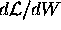is plotted. 2-D differential luminosity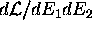is not plotted because the TopDrawer available at KEK HP station is not capable of 3-D plot.

The charge distribution and the beam field data for beam-beam interaction are computed at each time step for each longitudinal slice but they are not kept in the memory. They can be plotted only at the time moment and for the slice which is being proccessed. Thus, this command is to be inserted during PUSH loop. The slice is specified by the S operand.

PLOT BBFIELD, S=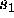S=(,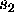,), [FILE=,] ;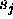Define the s-coordinate. Plot for the slice which contains one of's. Upto 5's can be specified.Next: CLEAR Up: Commands Previous: WRITEPRINT

Toshiaki Tauchi
Thu Dec 3 17:27:26 JST 1998